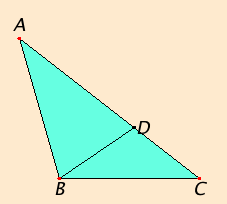# Proposition 18

In any triangle the angle opposite the greater side is greater.

Let ABC be a triangle having the side AC greater than AB.I say that the angle ABC is also greater than the angle BCA.

Since AC is greater than AB, make AD equal to AB, and join BD.

I.16

Since the angle ADB is an exterior angle of the triangle BCD, therefore it is greater than the interior and opposite angle DCB.

I.5

But the angle ADB equals the angle ABD, since the side AB equals AD, therefore the angle ABD is also greater than the angle ACB. Therefore the angle ABC is much greater than the angle ACB.

Therefore in any triangle the angle opposite the greater side is greater.

Q.E.D.

## Guide

#### On word order

In this translation of Euclid’s Elements the order of the words differs from the original Greek. In each of Euclid’s Greek sentences, the data, that is the geometric objects given or already constructed, appear first, and the remaining geometric objects appear later. This is possible in Greek since it is an inflected language and the word order is very flexible. On the other hand, the word order in English is intrinsic to the syntax and semantics of the sentence and is not very flexible.

Take, for instance, the statements of this and the next proposition. Very literal translations of these are (I.18) “In any triangle, the greater side [as subject] the greater angle [as object] subtends,” and (I.19) “In any triangle, the greater angle [as object] the greater side [as subject] subtends.”

Heath keeps the word order in his translation but makes the second statement passive: (I.18) “In any triangle the greater side subtends the greater angle,” and (I.19) “In any triangle the greater angle is subtended by the greater side.” Without the understanding that the data come first, these two sentences are logically equivalent.

In this translation the original word order is abandoned in order to make for more readable sentences and to clarify the meaning. Thus, (I.18) “In any triangle the angle opposite the greater side is greater,” and (I.19) “In any triangle the side opposite the greater angle is greater.”

It may sound like these two propositions really do say the same thing, but they don’t. they’re actually disguised converses of each other. I.18 says

if side AC > side AB, then angle ABC > angle BCA,

while I.19 says

if angle ABC > angle BCA, then side AC > side AB.

#### Use of Proposition 18

This proposition is used in the proof of proposition I.19.